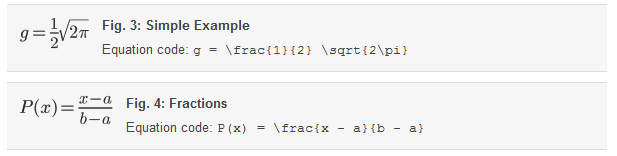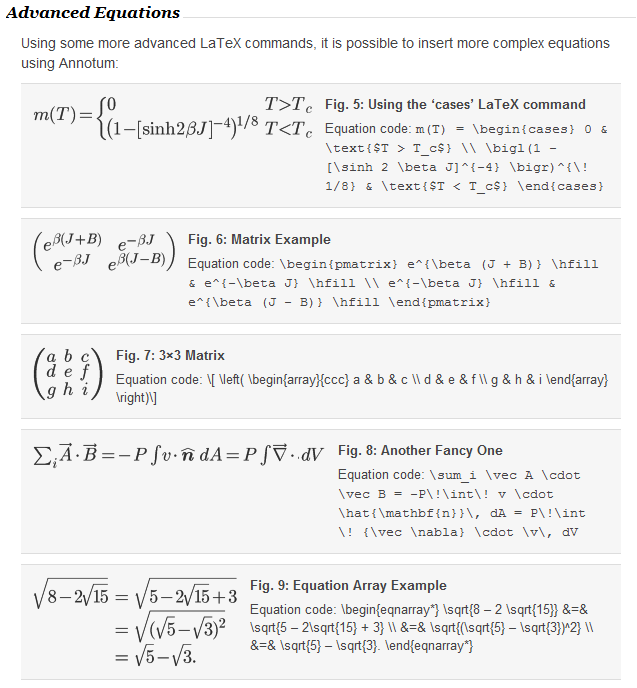# Equation Creation

Annotum supports equations using a built in equation editor. You can use the graphical controls to select equation items, or simply paste in your LaTeX equations.

## Inserting Equations

You can insert two kinds of equations: inline and figure.

### Inline Equations

The simplest equations are those included inline, that is, within the main text. For example, we can use the LaTeX code \sqrt{2\pi} to insert the following inline equation:.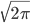You may notice that this is a bit larger than the default text, so the \small{} LaTeX command can be used to make a smaller inline equation:.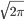To insert an inline equation, click the (Equations) toolbar button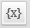, and fill in the Insert Equation dialog box: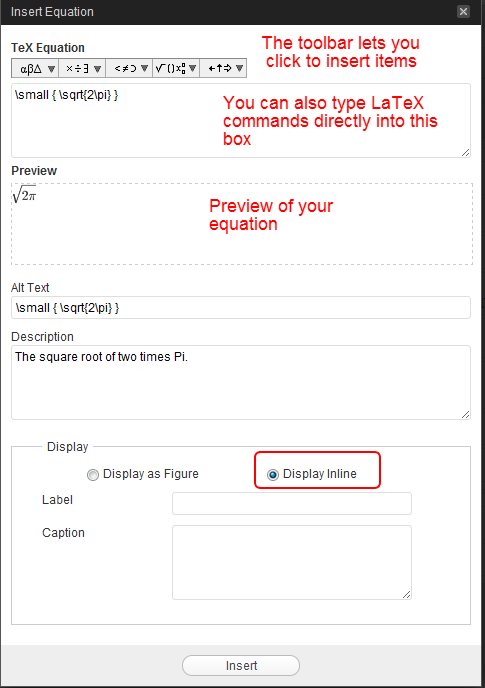#### Figure 1: The Insert Equation Dialog – Inline

Use this dialog box with the “Display Inline” option to insert an equation inline. You can use the equation toolbar to insert LaTeX code for the equation, or simply type it into the appropriate box. A preview area shows you how your equation will appear in your article.

### Equations as Figures

You can also insert equations as a figure. For that, use the “Display as Figure” option, and include a Label and Caption: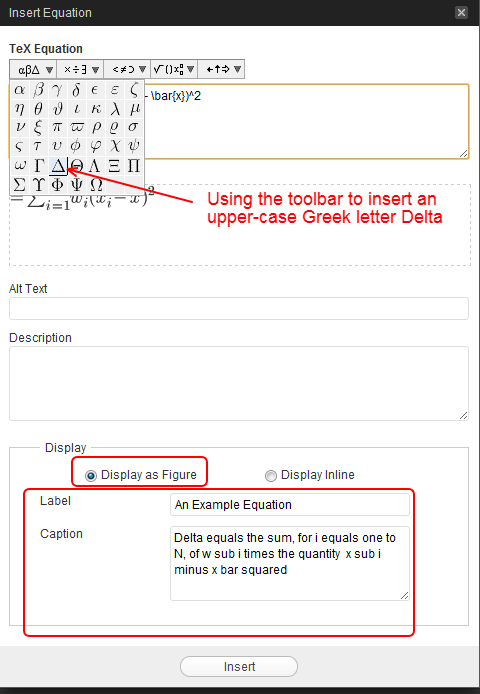#### Fig. 2: The Insert Equation Dialog – Figure

This figure shows how to insert an equation as a figure. The equation toolbar is being used to insert part of the equation.

### Simple Equations

Here are some simple equation examples. In all cases the LaTeX code used to create the equation is displayed in the Equation Caption. The examples presented here are based on these sample equations from Harvey Gould of Clark University.